Function Repository Resource:

# EarthquakeMagnitudeConvert

Convert between different earthquake magnitude scales

Contributed by: Jason Martinez
 ResourceFunction["EarthquakeMagnitudeConvert"][mag,scale1→scale2] converts earthquake magnitude mag from scale1 to scale2.

## Details and Options

The magnitude specification should be a real-valued number.
Supported magnitude scales can be obtained via ResourceFunction["EarthquakeMagnitudeConvert"]["Properties"].
Using All as the scale2 argument returns all possible converted values.

## Examples

### Basic Examples (2)

Discover equivalent values for an earthquake magnitude in different measurement systems:

 In:=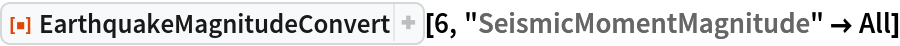Out=Convert between two systems of measurement:

 In:=Out=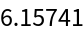### Scope (3)

Find supported magnitude scales:

 In:=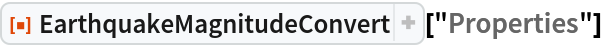Out=Learn the equivalent values for other earthquake magnitude measurements:

 In:=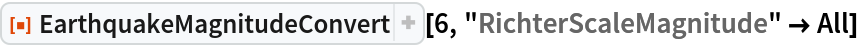Out=Explore how different magnitude scales relate:

 In:=Out=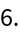### Applications (1)

Compare how the different scales differ in magnitude:

 In:=Out=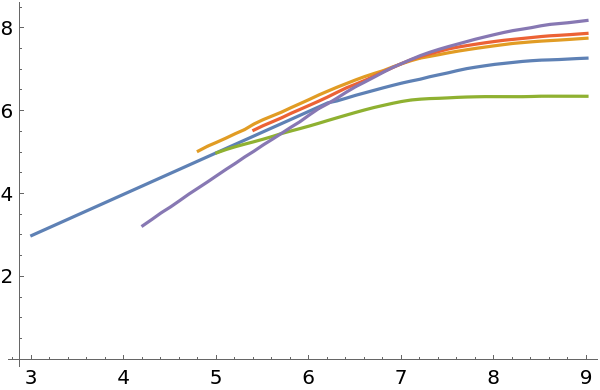### Possible Issues (1)

Magnitude scales are not supported for all possible values:

 In:=Out=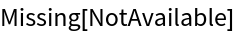## Version History

• 1.0.0 – 29 September 2020